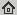# iPiano: Inertial Proximal Algorithm for Non-convex Optimization

SIAM Journal on Imaging Sciences, 7(2): 1388-1419, 2014
Abstract: In this paper we study an algorithm for solving a minimization problem composed of a differentiable (possibly non-convex) and a convex (possibly non-differentiable) function. The algorithm combines forward-backward splitting with an inertial force. A rigorous analysis of the algorithm for the proposed class of problems yields global convergence of the function values and the arguments. This makes the algorithm robust for usage on non-convex problems. The convergence result is obtained based on the Kurdyka-Lojasiewicz inequality. This is a very weak restriction, which was used to prove convergence for several other gradient methods. Furthermore, a convergence rate is established for the general problem class. In the more specialized case of convex functions, the optimal convergence rate is shown if one of the functions is stronlgy convex. We demonstrate iPiano on several computer vision problems, among them learned priors in denoising and optical flow estimation, and image compression.

## BibTex reference

```@Article{OB14,
author       = "P. Ochs and Y. Chen and T. Brox and T. Pock",
title        = "iPiano: Inertial Proximal Algorithm for Non-convex Optimization",
journal      = "SIAM Journal on Imaging Sciences",
number       = "2",
volume       = "7",
pages        = "1388-1419",
year         = "2014",
url          = "http://lmb.informatik.uni-freiburg.de/Publications/2014/OB14"
}
```